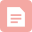import matplotlib.pyplot as plt
import numpy as np
import random

%matplotlib inline
%config InlineBackend.figure_format = ‘retina’

import string
characters = string.digits + string.ascii_uppercase
print(characters)

width, height, n_len, n_class = 170, 80, 4, len(characters)

random_str = ‘’.join([random.choice(characters) for j in range(4)])
img = generator.generate_image(random_str)

plt.imshow(img)
plt.title(random_str)X
X 的形状是 (batch_size, height, width, 3)，比如一批生成32个样本，图片宽度为170，高度为80，那么形状就是 (32, 80, 170, 3)，取第一张图就是 X。

y
y 的形状是四个 (batch_size, n_class)，如果转换成 numpy 的格式，则是 (n_len, batch_size, n_class)，比如一批生成32个样本，验证码的字符有36种，长度是4位，那么它的形状就是4个 (32, 36)，也可以说是 (4, 32, 36)，解码函数在下个代码块。

def gen(batch_size=32):
X = np.zeros((batch_size, height, width, 3), dtype=np.uint8)
y = [np.zeros((batch_size, n_class), dtype=np.uint8) for i in range(n_len)]
while True:
for i in range(batch_size):
random_str = ‘’.join([random.choice(characters) for j in range(4)])
X[i] = generator.generate_image(random_str)
for j, ch in enumerate(random_str):
y[j][i, :] = 0
y[j][i, characters.find(ch)] = 1
yield X, y

def decode(y):
y = np.argmax(np.array(y), axis=2)[:,0]
return ‘’.join([characters[x] for x in y])

X, y = next(gen(1))
plt.imshow(X)
plt.title(decode(y))

from keras.models import *
from keras.layers import *

input_tensor = Input((height, width, 3))
x = input_tensor
for i in range(4):
x = Convolution2D(322**i, 3, 3, activation=‘relu’)(x)
x = Convolution2D(32
2**i, 3, 3, activation=‘relu’)(x)
x = MaxPooling2D((2, 2))(x)

x = Flatten()(x)
x = Dropout(0.25)(x)
x = [Dense(n_class, activation=‘softmax’, name=‘c%d’%(i+1))(x) for i in range(4)]
model = Model(input=input_tensor, output=x)

model.compile(loss=‘categorical_crossentropy’,
metrics=[‘accuracy’])

from keras.utils.visualize_util import plot
from IPython.display import Image

plot(model, to_file=“model.png”, show_shapes=True)
Image(‘model.png’)

brew install graphviz
pip install pydot-ngmodel.fit_generator(gen(), samples_per_epoch=51200, nb_epoch=5,
nb_worker=2, pickle_safe=True,
validation_data=gen(), nb_val_samples=1280)

X, y = next(gen(1))
y_pred = model.predict(X)
plt.title(‘real: %s\npred:%s’%(decode(y), decode(y_pred)))
plt.imshow(X, cmap=‘gray’)from tqdm import tqdm
def evaluate(model, batch_num=20):
batch_acc = 0
generator = gen()
for i in tqdm(range(batch_num)):
X, y = next(generator)
y_pred = model.predict(X)
y_pred = np.argmax(y_pred, axis=2).T
y_true = np.argmax(y, axis=2).T
batch_acc += np.mean(map(np.array_equal, y_true, y_pred))
return batch_acc / batch_num

evaluate(model)

CTC Lossy_pred 是模型的输出，是按顺序输出的37个字符的概率，因为我们这里用到了循环神经网络，所以需要一个空白字符的概念；
labels 是验证码，是四个数字；
input_length 表示 y_pred 的长度，我们这里是15；
label_length 表示 labels 的长度，我们这里是4。
from keras import backend as K

def ctc_lambda_func(args):
y_pred, labels, input_length, label_length = args
y_pred = y_pred[:, 2:, :]
return K.ctc_batch_cost(labels, y_pred, input_length, label_length)

from keras.models import *
from keras.layers import *
rnn_size = 128

input_tensor = Input((width, height, 3))
x = input_tensor
for i in range(3):
x = Convolution2D(32, 3, 3, activation=‘relu’)(x)
x = Convolution2D(32, 3, 3, activation=‘relu’)(x)
x = MaxPooling2D(pool_size=(2, 2))(x)

conv_shape = x.get_shape()
x = Reshape(target_shape=(int(conv_shape), int(conv_shape*conv_shape)))(x)

x = Dense(32, activation=‘relu’)(x)

gru_1 = GRU(rnn_size, return_sequences=True, init=‘he_normal’, name=‘gru1’)(x)
gru_1b = GRU(rnn_size, return_sequences=True, go_backwards=True,
init=‘he_normal’, name=‘gru1_b’)(x)
gru1_merged = merge([gru_1, gru_1b], mode=‘sum’)

gru_2 = GRU(rnn_size, return_sequences=True, init=‘he_normal’, name=‘gru2’)(gru1_merged)
gru_2b = GRU(rnn_size, return_sequences=True, go_backwards=True,
init=‘he_normal’, name=‘gru2_b’)(gru1_merged)
x = merge([gru_2, gru_2b], mode=‘concat’)
x = Dropout(0.25)(x)
x = Dense(n_class, init=‘he_normal’, activation=‘softmax’)(x)
base_model = Model(input=input_tensor, output=x)

labels = Input(name=‘the_labels’, shape=[n_len], dtype=‘float32’)
input_length = Input(name=‘input_length’, shape=, dtype=‘int64’)
label_length = Input(name=‘label_length’, shape=, dtype=‘int64’)
loss_out = Lambda(ctc_lambda_func, output_shape=(1,),
name=‘ctc’)([x, labels, input_length, label_length])

model = Model(input=[input_tensor, labels, input_length, label_length], output=[loss_out])def gen(batch_size=128):
X = np.zeros((batch_size, width, height, 3), dtype=np.uint8)
y = np.zeros((batch_size, n_len), dtype=np.uint8)
while True:
for i in range(batch_size):
random_str = ‘’.join([random.choice(characters) for j in range(4)])
X[i] = np.array(generator.generate_image(random_str)).transpose(1, 0, 2)
y[i] = [characters.find(x) for x in random_str]
yield [X, y, np.ones(batch_size)*int(conv_shape-2),
np.ones(batch_size)*n_len], np.ones(batch_size)

def evaluate(model, batch_num=10):
batch_acc = 0
generator = gen()
for i in range(batch_num):
[X_test, y_test, _, _], _ = next(generator)
y_pred = base_model.predict(X_test)
shape = y_pred[:,2:,:].shape
ctc_decode = K.ctc_decode(y_pred[:,2:,:],
input_length=np.ones(shape)*shape)
out = K.get_value(ctc_decode)[:, :4]
if out.shape == 4:
batch_acc += ((y_test == out).sum(axis=1) == 4).mean()
return batch_acc / batch_num

from keras.callbacks import *
class Evaluate(Callback):
def init(self):
self.accs = []

def on_epoch_end(self, epoch, logs=None):
acc = evaluate(base_model)*100
self.accs.append(acc)
print
print 'acc: %f%%'%acc


evaluator = Evaluate()

model.fit_generator(gen(), samples_per_epoch=51200, nb_epoch=100,
callbacks=[evaluator],
nb_worker=2, pickle_safe=True)characters2 = characters + ’ ’
[X_test, y_test, _, _], _ = next(gen(1))
y_pred = base_model.predict(X_test)
y_pred = y_pred[:,2:,:]
out = K.get_value(K.ctc_decode(y_pred, input_length=np.ones(y_pred.shape)*y_pred.shape, ))[:, :4]
out = ‘’.join([characters[x] for x in out])
y_true = ‘’.join([characters[x] for x in y_test])

plt.imshow(X_test.transpose(1, 0, 2))
plt.title(‘pred:’ + str(out) + '\ntrue: ’ + str(y_true))

argmax = np.argmax(y_pred, axis=2)
list(zip(argmax, ‘’.join([characters2[x] for x in argmax])))random_str = ‘O0O0’
X = generator.generate_image(random_str)
X = np.expand_dims(X, 0)

y_pred = model.predict(X)
plt.title(‘real: %s\npred:%s’%(random_str, decode(y_pred)))
plt.imshow(X, cmap=‘gray’)《腾讯防水墙滑动拼图验证码》
《百度旋转图片验证码》
《网易易盾滑动拼图验证码》
《顶象区域面积点选验证码》
《顶象滑动拼图验证码》
《极验滑动拼图验证码》
《验证码终结者-基于CNN+BLSTM+CTC的训练部署套件》

1 应用AI立体防御技术，无需图形验证，彻底解决“安全”与“用户体验”的矛盾，互联网产品专注用户体验，无需为安全让步。
2 丰富可视化图表，防御拦截数据尽收眼底，实时查看当日数据详情与近期风险趋势。
3 SAAS极速接入，本地部署运行，毫秒级响应。交易风控引擎浓缩10M安装包，极速采集基础数据，匹配多维度风险特征。避免“云模式”网络延时问题。博客
YOLOX目标检测之入门实战-win10+cpu运行YOLOX
10-243万+博客

08-162935博客

03-176万+博客

03-081052博客

03-057万+博客

03-047万+博客

03-017万+博客

03-017万+博客

02-237万+博客

02-237万+博客

02-2112万+博客

02-197万+博客

02-181512博客

02-1820万+博客

02-162万+博客
「2021年」国内主流短信验证码平台综合评测
02-101万+博客
2021最新短信验证码接收平台收费价格对比「二」：亿佰云、秒嘀科技、极光短信、华信云通信
02-102万+博客

02-097万+博客

02-085532博客

02-082308

### “相关推荐”对你有帮助么？

•非常没帮助
•没帮助
•一般
•有帮助
•非常有帮助被折叠的  条评论 为什么被折叠?到【灌水乐园】发言点击重新获取扫码支付1.余额是钱包充值的虚拟货币，按照1:1的比例进行支付金额的抵扣。
2.余额无法直接购买下载，可以购买VIP、付费专栏及课程。余额充值